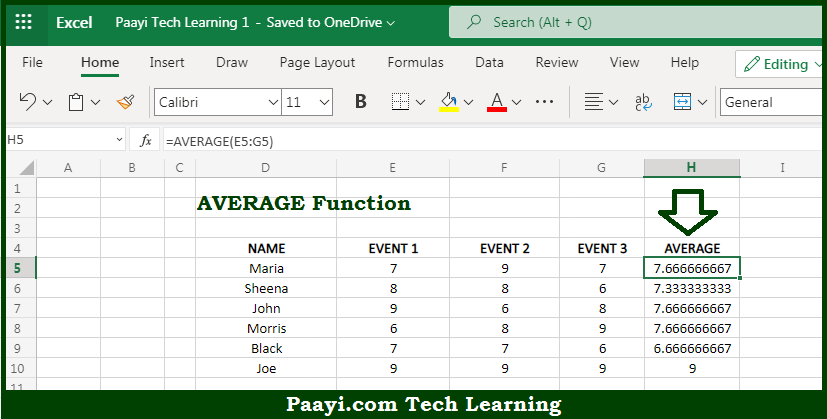# Learn How to Use Microsoft Excel AVERAGE Function

Written by | 0 Comments | 491 Views

In this article, you will learn how to use the Microsoft Excel AVERAGE function and its prime function in Microsoft Excel. You will also get to know the Microsoft Excel AVERAGE function return value and syntax with the help of some examples.

Microsoft Excel AVERAGE Function

The main purpose of the Microsoft Excel AVERAGE function is to get the average of a group of numbers. That implies, with the help of the AVERAGE function you can able to calculate the average or arithmetic mean of the group of numbers provided. It should be noted that the AVERAGE function can handle 255 individual arguments, that include numbers, cell references, ranges, arrays, and constants. So, with the help of the AVERAGE function, you can able to get the average of a group of numbers provided.

Return Value of AVERAGE Function

The return value will be the number representing an average.

Syntax of AVERAGE Function

=AVERAGE(number1, [number2], ...)

Where the arguments:

• number1: This is the number or cell reference that refers to the numeric value.
• number2: This is the second number or cell reference that refers to the numeric value (Optional).

## How to Use Microsoft Excel AVERAGE Function?So we know that Microsoft Excel AVERAGE function you can able to to to get the average of the group of numbers. That implies, with the help of the AVERAGE function you can able to calculate the average or arithmetic mean of the group of numbers provided. It should be noted that the AVERAGE function can handle 255 individual arguments, that include numbers, cell references, ranges, arrays, and constants. So, with the help of the AVERAGE function, you can able to get the average of the group of numbers provided.